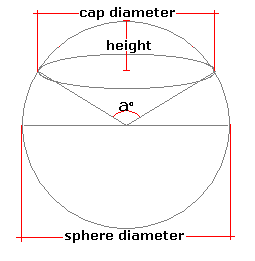Spike's Calculators

# Spherical Cap Volume and Surface AreaCalculate the volume, arc length, base and surface area of a spherical cap.

This calculator works for any measurement unit. For example, if you calculate inches, inches will have to be used in all the length fields, and the result of the areas will be square inches; the volume results in cubic inches and length measurements in the starting unit inches.

### Spherical Cap

Height of Cap #
Base Lenght of Cap #
Decimal Precision #

#### Results:

 Diameter of the Sphere # Volume of the Cap #³ Bottom Area #² Cap Area #² Surface Area #² Internal Angle ° Arc Length #

#### Calculation

• the height of the cap
• the base length of the cap
• decimal precision, number of digits after the decimal point

#### Results

• the diameter of the sphere (starting unit)
• sphere cap volume (unit cubed)
• the base (bottom) area of the cap (unit squared)
• the cap (calotte area) area (unit squared)
• the surface area (unit squared)
• the internal angle (can not be more than 180°)
• arc length of the cap (starting unit)
##### Formula
```R= ((C/2)²/H+H)/2
V= H²Π/3(3R-H)
B =Π(C/2)²
S = 2ΠRH
SA = B+S
A = RΠa
s/v = SA/V
where R is the radius of the sphere
C the base length of the cap
H the height
Π = 3.14159265
V the volume
B the area of the base
S the surface area of the cap minus base
SA the surface area
A the length of the arc
s/v is the surface area to volume ratio
```